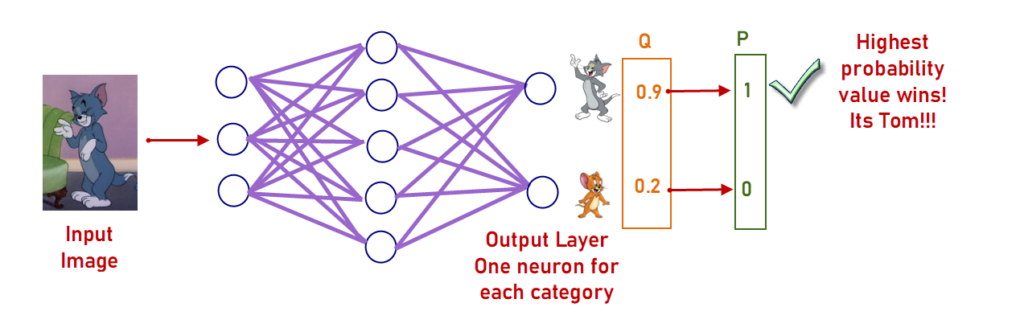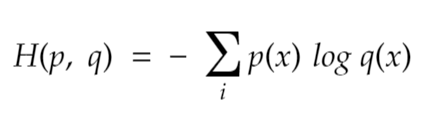# What are the Loss Functions for a Deep Learning model?

Loss: The error value received in 1 iteration

Cost: The sum of squares of all the errors, received from each iteration

### Loss Function in Neural Network:

Neural Network works in an iterative manner to get the optimum value for weights.

For each iteration, a specific loss function is used to evaluate the error value. This loss function tells the model on how the errors should be calculated.

We know that there are two types of data that we need to predict.

1. Continuous data (example: price, score)
2. Categorical/Nominal value (example: Yes/No, Dog/Cat, Identify a digit, Identify the objects)

The continues value is a numerical value that the model gives as an output from forward propagation. For example, consider we are sending 1 input data into the network for an iteration. If this is a price value & the model gives the training value as \$100 but the original value is \$120, then the error is \$120 – \$100 = \$20. We can easily check the error value.

But in categorical value, we cannot apply the same method. We can only say how many predictions were correct and how many were wrong.

So we have numerous ways to find the loss value for an iteration. In this article, lets see what are the most commonly used Loss Functions for a NN.

### Loss Functions for Continuous Values:

• MeanSquaredError class:
• Computes the Mean of Square of the differences of true and predicted value of all the input data in 1 iteration.
• loss = Sum(Square(true_value – predicted))/number of inputs
• The reason we take square is to convert the negative difference to the positive value.
• Usage:
• MeanAbsoluteError class
• Computes the Mean of absolute of the differences of true and predicted value of all the input data in 1 iteration.
• loss = Sum(Absolute(true_value – predicted))/number of inputs
• This loss function is similar to the previous once but instead of square, we just ignore the negative symbol.
• Usage:
• MeanAbsolutePercentageError class:
• It check how many percentage in total input data gets the correct prediction.
• Percentage of the error is
• loss = Sum((Absolute(true_value – predicted)/true_value) * 100)/number of inputs
• Usage:

### Loss Functions for Categorical Values:

To identify objects from Images in Neural Network, we use Softmax function in the last layer. This Softmax function given the estimated probability value for each category.

We know we take the probability value as 1 if it is >0.5 and 0 if it is < 0.5.

#### How can we find the loss value here?

To evaluate the loss value from the probability value given by Softmax, we use cross-entropy.

Cross entropy takes the log of each probability value of each data and finds the mean of all in the iteration. This value is the cross entropy value.Image by Author

In the above example, we are identifying whether it is Tom or Jerry based on 3 input features.

The Output layer gives the probability (Q) of 0.9 as Tom and 0.2 as Jerry [0.9, 0.2]. The True one-hot encoded target variable’s value for this data is [1, 0] . Even though 0.9 is very near to 1, it is not. This difference 0.1 and 0.2 value receive as Jerry is the loss we got for this data.

Cross entropy finds this loss using the below equation.Cross Entropy Equation

For the above example, for the estimated probability [0.9, 0.2], the cross entropy value of this data is calculated below.

So, the total cross entropy value for this data is 0.10.

If the iteration is a batch of 50 data points, then the mean of cross entropy value for all the data points in the batch is taken as over all cross entropy value of that iteration, which is the loss.

The lesser the Cross Entropy, better the model.

Depending upon this loss value, the weights of the model will be adjusted for the next iteration.

To use cross entropy in our Deep Learning Models, we have a set of loss functions readily available from Keras.

• BinaryCrossentropy class
• As the name says, we can use this loss function when there are only 2 classes True and False (1 and 0).
• Usage:
• CategoricalCrossentropy class
• We can use this loss function when there are two or more output categories. Example: Cat/Dog/Rabbit
• This function uses the one-hot encoded array.
• If the Output is [1,0,0] then it means, the output is first category.
• If the Output is [0,1,0] then the output is of Second category and so on.

Usage:

• SparseCategoricalCrossentropy class
• We can handle the two or more categorical values in two ways:
• Encode the label values using One-Hot encoding or Categorical encoding
• Encode the label values as Integers.
• This loss function can be used for two or more categories but the classes if it is encoded as Integers (i.e. mutually exclusive – The model won’t care about the probability of other classes.)
• One major difference between CategoricalCrossentropy and SparseCategoricalCrossentropy is, this loss function takes input as integers. If the values are one-hot encoded, then we must use only CategoricalCrossentropy.
• The final output layer’s code could be as below:

Notice that the Dense count is “no_classes”. i.e. the output layer gives only one integer value.

Mention the loss function as below to compile the model.

• Poisson class
• We use Poisson loss if the Output data is from a Poisson distribution.

Usage:

• KLDivergence class
• The KLDivergence is used to measure the difference between two estimated probabilities.
• The loss value calculation for this loss function is loss = y_true * log(y_true / y_pred)
• This looks similar to Cross Entropy but it is not.
• The Equation of KLDivergence is given below:

Usage:

0